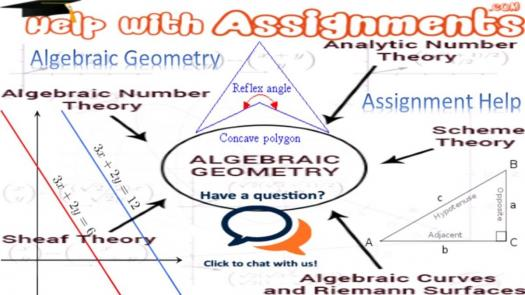# Algebraic Geometry Test

10 Questions | Total Attempts: 125SettingsConsidered boring by a lot of people, algebraic geometry is another topic in mathematics that's concerned with the zeros of multivariate polynomials. The world over, contemporary mathematicians use commutative algebra to answer geometrical problems. If your discipline applies this mathematical concept, then give it a try.

Related Topics
• 1.
Examples of plane algebraic curves studied in algebraic geometry are these except
• A.

Hyperbolas

• B.

Parabolas

• C.

Cones

• D.

Ellipses

• 2.
Which of the following is not of concern in algebraic geometry?
• A.

Singular points

• B.

Inflection points

• C.

Points at infinity

• D.

Central points

• 3.
Algebraic geometry is related to the following except
• A.

Number theory

• B.

Complex analysis

• C.

Topology

• D.

Computational analysis

• 4.
The development of algebraic geometry can be traced to
• A.

5th century BC

• B.

7th century BC

• C.

11 century BC

• D.

3 century BC

• 5.
Which of the following mathematicians are not associated with algebraic geometry?
• A.

Menaechmus

• B.

Archimedes

• C.

Pythagoras

• D.

Apollonius

• 6.
Algebraic geometry is applied in the following fields except
• A.

Robotics

• B.

Phylogenetics

• C.

Geometric modelling

• D.

Structural engineering

• 7.
A polynomial vanishes  at a certain point when it's evaluations
• A.

Less than zero

• B.

Greater than zero

• C.

Is zero

• D.

Has an element of zero

• 8.
When an algebraic set can not be expressed as a union of two smaller algebraic sets, it is said to be
• A.

Reducible

• B.

Irreducible

• C.

Perfect

• D.

Imperfect

• 9.
What is an irreducible algebraic set called?
• A.

Prime deal

• B.

A notation

• C.

Variety

• D.

Regular function

• 10.
Who presented an algorithm for solving systems of homogeneous polynomial equations with computational complexity?
• A.

George Collins

• B.

Daniel Lazard

• C.

Bruno Buchberger

• D.

Gilbert Macaulay